# 3.1 Springs and particles

The most basic type of mechanical model consists simply of particles connected together by axial springs. Particles are implemented by the class Particle, which is a dynamic component containing a three-dimensional position state, a corresponding velocity state, and a mass. It is an instance of the more general base class Point, which is used to also implement spatial points such as markers which do not have a mass.

## 3.1.1 Axial springs and materials

An axial spring is a simple spring that connects two points and is implemented by the class AxialSpring. This is a force effector component that exerts equal and opposite forces on the two points, along the line separating them, with a magnitude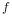that is a function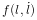of the distance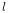between the points, and the distance derivative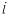.

Each axial spring is associated with an axial material, implemented by a subclass of AxialMaterial, that specifies the function. The most basic type of axial material is a LinearAxialMaterial, which determinesaccording to the linear relationship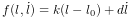(3.1)

where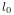is the rest length and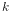and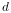are the stiffness and damping terms. Bothandare properties of the material, whileis a property of the spring.

Axial springs are assigned a linear axial material by default. More complex, nonlinear axial materials may be defined in the package artisynth.core.materials. Setting or querying a spring’s material may be done with the methods setMaterial() and getMaterial().

## 3.1.2 Example: a simple particle-spring model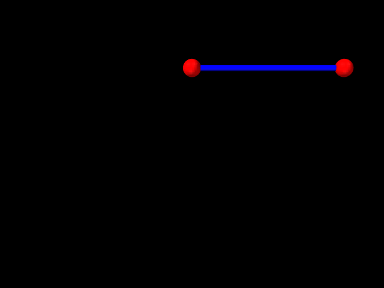Figure 3.1: ParticleSpring model loaded into ArtiSynth.

An complete application model that implements a simple particle-spring model is given below.

1 package artisynth.demos.tutorial;
2
3 import java.awt.Color;
4 import maspack.matrix.*;
5 import maspack.render.*;
6 import artisynth.core.mechmodels.*;
7 import artisynth.core.materials.*;
8 import artisynth.core.workspace.RootModel;
9
10 /**
11  * Demo of two particles connected by a spring
12  */
13 public class ParticleSpring extends RootModel {
14
15    public void build (String[] args) {
16
17       // create MechModel and add to RootModel
18       MechModel mech = new MechModel ("mech");
20
21       // create the components
22       Particle p1 = new Particle ("p1", /*mass=*/2, /*x,y,z=*/0, 0, 0);
23       Particle p2 = new Particle ("p2", /*mass=*/2, /*x,y,z=*/1, 0, 0);
24       AxialSpring spring = new AxialSpring ("spr", /*restLength=*/0);
25       spring.setPoints (p1, p2);
26       spring.setMaterial (
27          new LinearAxialMaterial (/*stiffness=*/20, /*damping=*/10));
28
29       // add components to the mech model
33
34       p1.setDynamic (false);                // first particle set to be fixed
35
36       // increase model bounding box for the viewer
37       mech.setBounds (/*min=*/-1, 0, -1, /*max=*/1, 0, 0);
38       // set render properties for the components
39       RenderProps.setSphericalPoints (p1, 0.06, Color.RED);
40       RenderProps.setSphericalPoints (p2, 0.06, Color.RED);
41       RenderProps.setCylindricalLines (spring, 0.02, Color.BLUE);
42    }
43 }

Line 1 of the source defines the package in which the model class will reside, in this case artisynth.demos.tutorial. Lines 3-8 import definitions for other classes that will be used.

The model application class is named ParticleSpring and declared to extend RootModel (line 13), and the build() method definition begins at line 15. (A no-args constructor is also needed, but because no other constructors are defined, the compiler creates one automatically.)

To begin, the build() method creates a MechModel named "mech", and then adds it to the models list of the root model using the addModel() method (lines 18-19). Next, two particles, p1 and p2, are created, with masses equal to 2 and initial positions at 0, 0, 0, and 1, 0, 0, respectively (lines 22-23). Then an axial spring is created, with end points set to p1 and p2, and assigned a linear material with a stiffness and damping of 20 and 10 (lines 24-27). Finally, after the particles and the spring are created, they are added to the particles and axialSprings lists of the MechModel using the methods addParticle() and addAxialSpring() (lines 30-32).

At this point in the code, both particles are defined to be dynamically controlled, so that running the simulation would cause both to fall under the MechModel’s default gravity acceleration of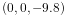. However, for this example, we want the first particle to remain fixed in place, so we set it to be non-dynamic (line 34), meaning that the physical simulation will not update its position in response to forces (Section 3.1.3).

The remaining calls control aspects of how the model is graphically rendered. setBounds() (line 37) increases the model’s “bounding box” so that by default it will occupy a larger part of the viewer frustum. The convenience method RenderProps.setSphericalPoints() is used to set points p1 and p2 to render as solid red spheres with a radius of 0.06, while RenderProps.setCylindricalLines() is used to set spring to render as a solid blue cylinder with a radius of 0.02. More details about setting render properties are given in Section 4.3.

To run this example in ArtiSynth, select All demos > tutorial > ParticleSpring from the Models menu. The model should load and initially appear as in Figure 3.1. Running the model (Section 1.5.3) will cause the second particle to fall and swing about under gravity.

## 3.1.3 Dynamic, parametric, and attached components

By default, a dynamic component is advanced through time in response to the forces applied to it. However, it is also possible to set a dynamic component’s dynamic property to false, so that it does not respond to force inputs. As shown in the example above, this can be done using the method setDynamic():

  comp.setDynamic (false);


The method isDynamic() can be used to query the dynamic property.

Dynamic components can also be attached to other dynamic components (as mentioned in Section 1.2) so that their positions and velocities are controlled by the master components that they are attached to. To attach a dynamic component, one creates an AttachmentComponent specifying the attachment connection and adds it to the MechModel, as described in Section 3.6. The method isAttached() can be used to determine if a component is attached, and if it is, getAttachment() can be used to find the corresponding AttachmentComponent.

Overall, a dynamic component can be in one of three states:

active

Component is dynamic and unattached. The method isActive() returns true. The component will move in response to forces.

parametric

Component is not dynamic, and is unattached. The method isParametric() returns true. The component will either remain fixed, or will move around in response to external inputs specifying the component’s position and/or velocity. One way to supply such inputs is to use controllers or input probes, as described in Section 5.

attached

Component is attached. The method isAttached() returns true. The component will move so as to follow the other master component(s) to which it is attached.

## 3.1.4 Custom axial materials

Application authors may create their own axial materials by subclassing AxialMaterial and overriding the functions

double computeF (l, ldot, l0, excitation);
double computeDFdl (l, ldot, l0, excitation);
double computeDFdldot (l, ldot, l0, excitation);
boolean isDFdldotZero ();

where excitation is an additional excitation signal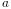, which is used to implement active springs and which in particular is used to implement axial muscles (Section 4.4), for whichis usually in the range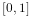.

The first three methods should return the values of(3.2)

respectively, while the last method should return true if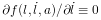; i.e., if it is always equals to 0.

## 3.1.5 Damping parameters

Mechanical models usually contain damping forces in addition to spring-type restorative forces. Damping generates forces that reduce dynamic component velocities, and is usually the major source of energy dissipation in the model. Damping forces can be generated by the spring components themselves, as described above.

A general damping can be set for all particles by setting the MechModel’s pointDamping property. This causes a force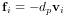(3.3)

to be applied to all particles, where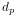is the value of the pointDamping and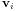is the particle’s velocity.

pointDamping can be set and queried using the MechModel methods

setPointDamping (double d);
double getPointDamping();

In general, whenever a component has a property propX, that property can be set and queried in code using methods of the form

  setPropX (T d);
T getPropX();


where T is the type associated with the property.

pointDamping can also be set for particles individually. This property is inherited (Section 1.4.3), so that if not set explicitly, it inherits the nearest explicitly set value in an ancestor component.